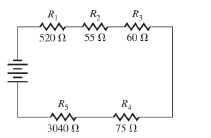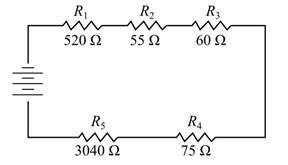Chapter 2, Problem 3CR

Chapter
Section
Textbook Problem

Find the total resistance in the series circuit in Illustration 2.ILLUSTRATION 2

To determine

To calculate: The total resistance in the series circuit shown below:Explanation

Given Information:

The provided figure is illustrated below:

Formula used:

In a series circuit the total resistance is given by:

Total resistance, RT=sum of all the resistances in the circuit

Calculation:

An electric circuit with only one path for the current, I, to flow is called a series circuit.

In a series circuit, the total resistance is equal to the sum of all the resistances in the circuit. Thus according to the given question,

Total resistance, RT=sum of all the resistances in the circuit=R1+R2+R3+R4+R5

Add all the resistances in the circuit

Still sussing out bartleby?

Check out a sample textbook solution.

See a sample solution

The Solution to Your Study Problems

Bartleby provides explanations to thousands of textbook problems written by our experts, many with advanced degrees!

Get Started

Find more solutions based on key concepts